# Singular function

(diff) ← Older revision | Latest revision (diff) | Newer revision → (diff)

A non-constant continuous function of bounded variation whose derivative is zero almost-everywhere on the interval on which it is defined. Singular functions arise as summands in the Lebesgue decomposition of functions of bounded variation. For example, every continuous functionof bounded variation on an intervalcan be uniquely written in the form of a sum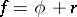, whereis an absolutely-continuous function (cf. Absolute continuity) with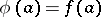andis a singular function or identically zero.

Example. Let. Anycan be written in the formwhere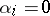,or 2 for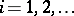. Thus, if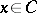, whereis the Cantor set, thenor 2 for all. Let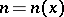be the first subscript for which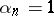; if there are no such subscripts, then one takes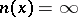. The function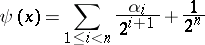is then a monotone singular function on.

How to Cite This Entry:
Singular function. Encyclopedia of Mathematics. URL: http://encyclopediaofmath.org/index.php?title=Singular_function&oldid=13838
This article was adapted from an original article by B.I. Golubov (originator), which appeared in Encyclopedia of Mathematics - ISBN 1402006098. See original article